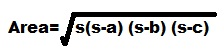# C++ Tutorial With Basic Programs for Beginners

C++ tutorial, C++ programs, C++ code, C++ flowcharts, C++ statements, C++ syntax, C++ examples

## Thursday, March 22, 2018

### Program Area of Triangle Algorithm Flowchart

Q: Write a C++ Program to input three sides of a triangle. The program then calculates the Area of triangle by the formulaFormula of area of triangle when 3 sides given
Where s = ( a+b+c ) / 2

## Write Down Algorithm of C++ Program Area of Triangle

1. Start
2. Input a,b,c
3. Calculate s = (a + b + c ) / 2
4. Calculate area = sqrt( s*(s-a)*(s-b)*(s-c))
5. print "Area of Triangle=", area
6. End

### Develop Flowchart of C++ Program Area of Triangledevelop flowchart c plus plus program area of triangle

Generally we prepare a flowchart and algorithm which is independent of  any programming language so here is a better flowchart version for C++ Program Area of Triangle with mathematical formula instead of C++ arithmetic expressions.

#### Flowchart for C++ Program How To Calculate Area of triangle when 3 side are inputdevelop flowchart c plus plus program area of triangle programming language independent

### Source code for modern C++ IDEs /Compilers ( Used Dev C++ )

```#include<iostream>
#include<math.h>

using namespace std;
int main( )
{
float a,b,c,s,area;

cout<<"Enter side A of triangle = ";
cin>>a;
cout<<"Enter side B of triangle = ";
cin>>b;
cout<<"Enter side C of triangle = ";
cin>>c;

s = (a + b + c ) / 2;

area = sqrt ( s * (s - a ) * (s - b ) * (s - c ) ) ;

cout<< "Area of Triangle = "<<area;
return 0;

}
```

### Output of the sample run:

Enter side A of triangle = 5
Enter side B of triangle = 5
Enter side C of triangle = 5
Area of Triangle = 10.8253
--------------------------------
Process exited after 4.697 seconds with return value 0
Press any key to continue . . .

### Write Down Source Code of C++ Program Area of Triangle for Turbo C++ 3.0 Compiler / IDE

#include<iostream.h>
#include<conio.h>
#include<math.h>
void main( )
{
float a,b,c,s,area;
clrscr();
cout<<"Enter side A of triangle = ";
cin>>a;
cout<<"Enter side B of triangle = ";
cin>>b;
cout<<"Enter side C of triangle = ";
cin>>c;

s = (a + b + c ) / 2;

area = sqrt ( s * (s - a ) * (s - b ) * (s - c ) ) ;
cout<< "Area of Triangle = "<<area;

getch();
}

Share:

#### 2 comments:

1.2.We Love To Hear From You!